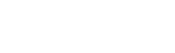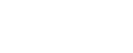# Minimal Detectable Difference

The minimal detectable difference is the smallest difference, or change, that can be statistically detected in a given study.

It  is  dependent on your desired significance level, and has been defined as the smallest difference δ where p (δ) ≤ α.

In clinical trials, the minimal detectable difference is the smallest difference between treatments that is medically significant. For a study of association (in which you’re researching how variables relate to each other) it is the minimum plausible change in dependent variable per change in the independent variable.

## Calculating Minimal Detectable Difference

There are many different formulas for calculating the minimal detectable difference, and which one you want to use will depend on your project. A version commonly used in biostatics is:Where n is the sample size and  σ 2 is the variance of the response variable. In this  formula z represents the z scores over the given intervals. 1- β is the statistical power.

Valk defined another simple formula for an experiment:Here, α is the significance level you are going for, β is 1 minus the statistical power, and  zα is the 100(1- α) z-score, the percentile of the standard normal distribution.  Also, as in the previous formula, n is the number of observations.

## Reasons to Find the Minimal Detectable Difference

The minimal detectable difference is important when planning experiments because it gives you the information you need to decide what equipment to use, how accurately to take measurements, and how frequently to sample. It also translates your desired significance level into terms that are relevant to your groundwork, and with it you can see the importance of each of your significant figures in real, concrete terms.

## Sources

CITE THIS AS:
Stephanie Glen. "Minimal Detectable Difference" From StatisticsHowTo.com: Elementary Statistics for the rest of us! https://www.statisticshowto.com/minimal-detectable-difference/
---------------------------------------------------------------------------Need help with a homework or test question? With Chegg Study, you can get step-by-step solutions to your questions from an expert in the field. Your first 30 minutes with a Chegg tutor is free!